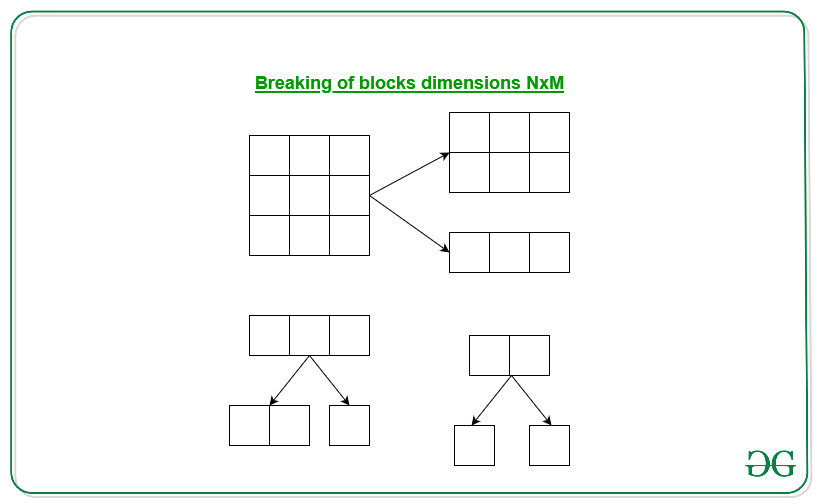# Identical Splitting in a rectangular grid

Given a rectangular grid NxM dimensions, the task is to find the minimum number of cuts required to break the given rectangular grid into a square of size 1×1.

Examples:

Input: N = 4, M = 4
Output: 15

Input: N = 2, M = 1
Output: 1

## Recommended: Please try your approach on {IDE} first, before moving on to the solution.

Approach:The above images shows the splitting of the rectangular grid. We can observe that every single cut increases the number of rectangles of different dimensions by 1. We will do the splitting untill we reach the square of dimension 1×1.

So for the given rectangular dimensions of NxM, the total number of squares of dimensions 1×1 is N*M. Therefore we required N*M – 1 cuts to break the given rectangular dimensions of NxM into squares of dimension 1×1.

Below is the implementation of the above approach:

## C++

 `// C++ program of the above approach ` `#include ` `using` `namespace` `std; ` ` `  `// Function to find the minimum cuts ` `void` `minimumCuts(``int` `N, ``int` `M) ` `{ ` ` `  `    ``// Print the minimum cuts using ` `    ``// the formula ` `    ``cout << (N * M - 1); ` `} ` ` `  `// Driver Code ` `int` `main() ` `{ ` ` `  `    ``// Given dimensions ` `    ``int` `N = 4, M = 4; ` ` `  `    ``// Function call ` `    ``minimumCuts(N, M); ` ` `  `    ``return` `0; ` `} `

## Java

 `// Java program of the above approach ` `import` `java.util.*; ` ` `  `class` `GFG{ ` ` `  `// Function to find the minimum cuts ` `static` `void` `minimumCuts(``int` `N, ``int` `M) ` `{ ` `     `  `    ``// Print the minimum cuts using ` `    ``// the formula ` `    ``System.out.print(N * M - ``1``); ` `} ` ` `  `// Driver Code ` `public` `static` `void` `main(String[] args) ` `{ ` `     `  `    ``// Given dimensions ` `    ``int` `N = ``4``, M = ``4``; ` ` `  `    ``// Function call ` `    ``minimumCuts(N, M); ` `} ` `} ` ` `  `// This code is contributed by Rohit_ranjan `

## Python3

 `# Python3 program of the above approach ` ` `  `# Function to find the minimum cuts ` `def` `minimumCuts(N, M): ` `     `  `    ``# Print the minimum cuts using ` `    ``# the formula ` `    ``print``(N ``*` `M ``-` `1``) ` ` `  `# Driver Code  ` `if` `__name__ ``=``=` `"__main__"``: ` `     `  `    ``# Given dimensions ` `    ``N ``=` `4` `    ``M ``=` `4` `     `  `    ``# Function call ` `    ``minimumCuts(N, M) ` `     `  `# This code is contributed by coder001 `

## C#

 `// C# program of the above approach ` `using` `System; ` ` `  `class` `GFG{ ` ` `  `// Function to find the minimum cuts ` `static` `void` `minimumCuts(``int` `N, ``int` `M) ` `{ ` `     `  `    ``// Print the minimum cuts using ` `    ``// the formula ` `    ``Console.Write(N * M - 1); ` `} ` ` `  `// Driver Code ` `public` `static` `void` `Main(String[] args) ` `{ ` `     `  `    ``// Given dimensions ` `    ``int` `N = 4, M = 4; ` ` `  `    ``// Function call ` `    ``minimumCuts(N, M); ` `} ` `} ` ` `  `// This code is contributed by Princi Singh `

Output:

```15
```

Time Complexity: O(1)

Attention reader! Don’t stop learning now. Get hold of all the important DSA concepts with the DSA Self Paced Course at a student-friendly price and become industry ready.

My Personal Notes arrow_drop_upIf you like GeeksforGeeks and would like to contribute, you can also write an article using contribute.geeksforgeeks.org or mail your article to contribute@geeksforgeeks.org. See your article appearing on the GeeksforGeeks main page and help other Geeks.

Please Improve this article if you find anything incorrect by clicking on the "Improve Article" button below.

Article Tags :
Practice Tags :

3

Please write to us at contribute@geeksforgeeks.org to report any issue with the above content.